# VOLUME What is Volume Volume is the measure

• Slides: 18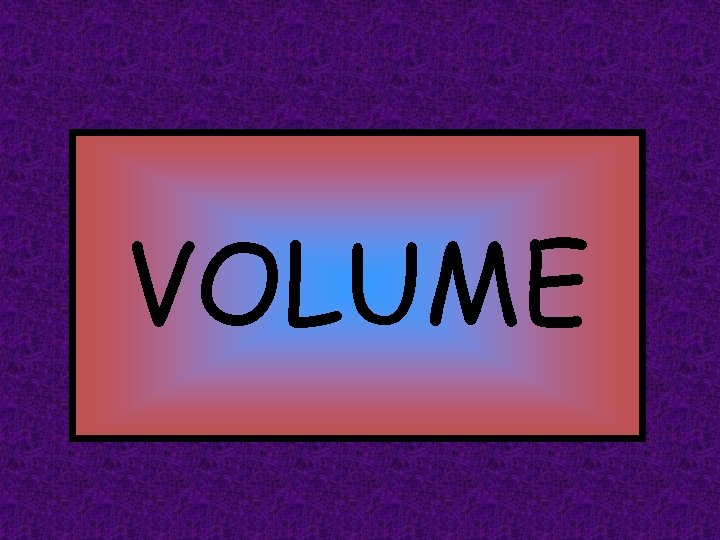VOLUME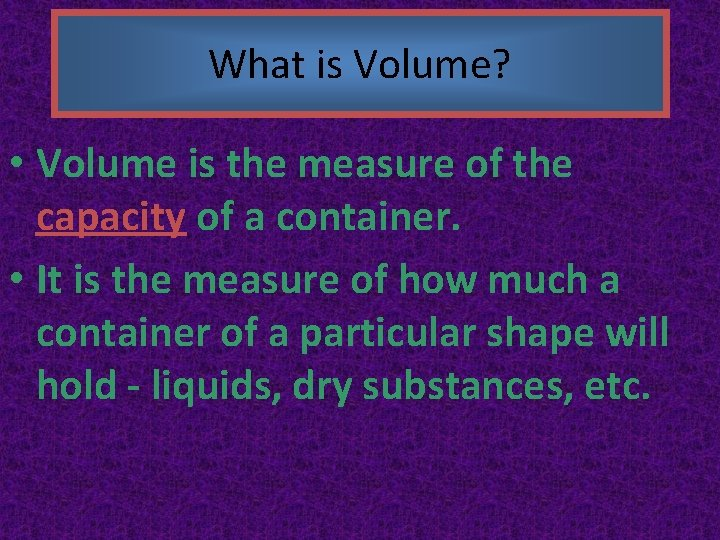What is Volume? • Volume is the measure of the capacity of a container. • It is the measure of how much a container of a particular shape will hold - liquids, dry substances, etc.VOLUME is the amount of liquid or solid that will FILL a 3 -Dimensional object! *Always measured in units cubed (u 3)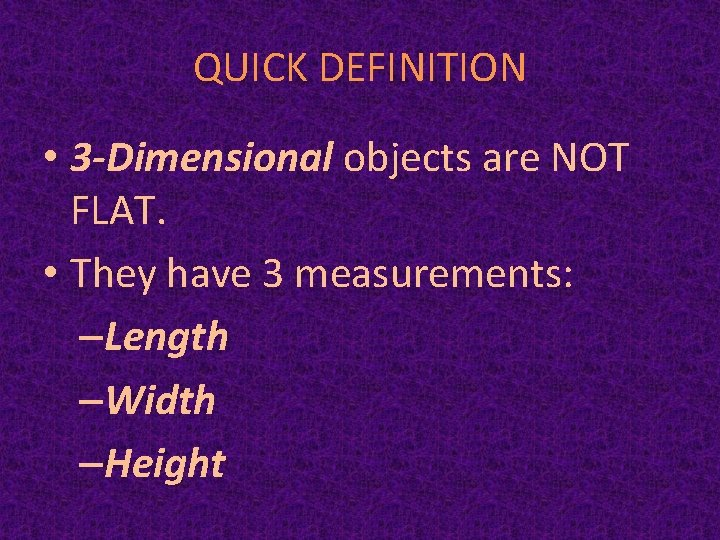QUICK DEFINITION • 3 -Dimensional objects are NOT FLAT. • They have 3 measurements: –Length –Width –HeightCubic Units ¤Volume is measured in cubic units. ¤Use cubes to fill a rectangular prism such as a box.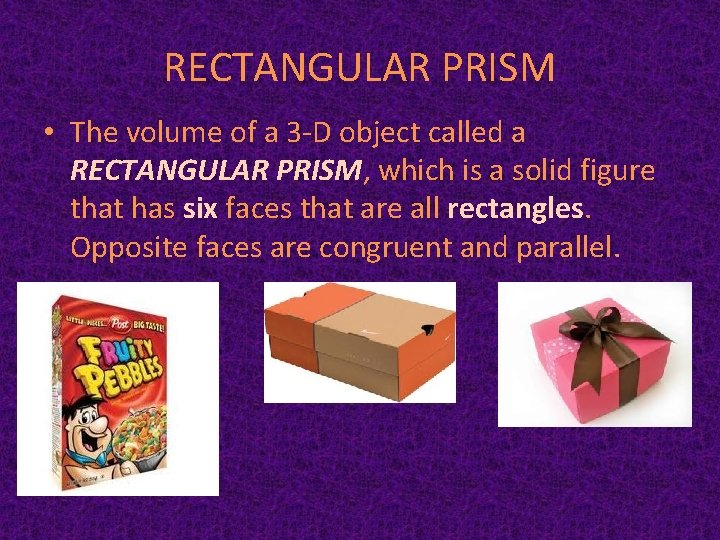RECTANGULAR PRISM • The volume of a 3 -D object called a RECTANGULAR PRISM, which is a solid figure that has six faces that are all rectangles. Opposite faces are congruent and parallel.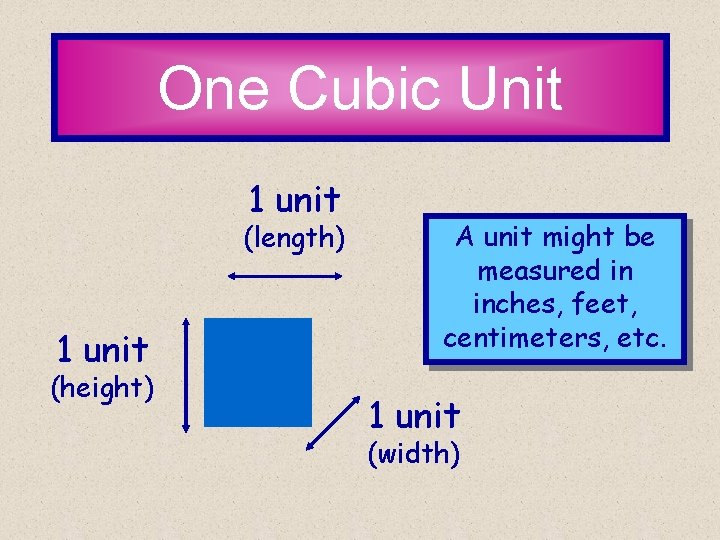One Cubic Unit 1 unit (length) 1 unit (height) A unit might be measured in inches, feet, centimeters, etc. 1 unit (width)How many cubic units is this rectangle? What did you find? Yes, it is 8 cubic units!What’s the formula? The formula for finding the volume of a rectangle is …. L x W x H = Volume This means we take the length times the width, then multiply that by the height.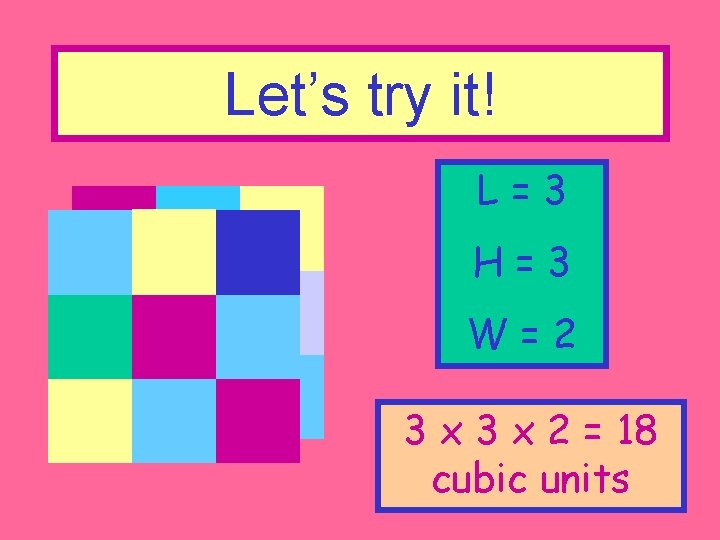Let’s try it! L=3 H=3 W=2 3 x 2 = 18 cubic unitsFind the volume of this one! What did you find? 4 x 2 x 3 = 24 cubic units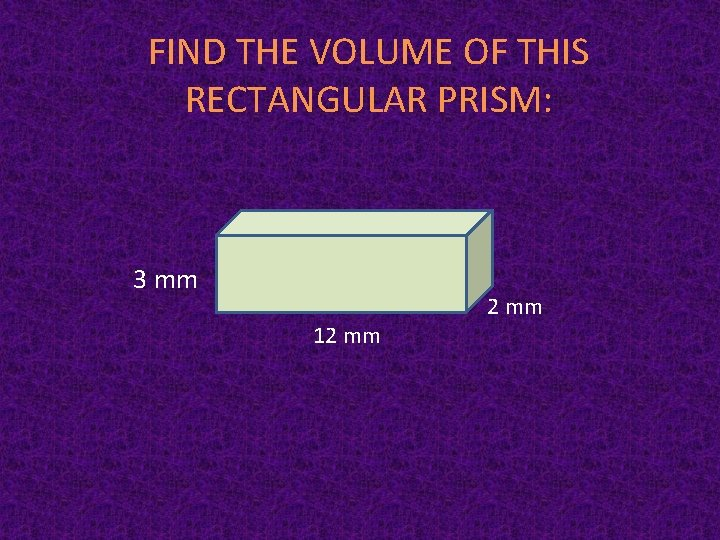FIND THE VOLUME OF THIS RECTANGULAR PRISM: 3 mm 2 mm 12 mmLet’s practice some problems and solving them. A cereal box has a length of 8 inches, a width of 1. 75 inches, and a height of 12. 125 inches. How much cereal will the box hold?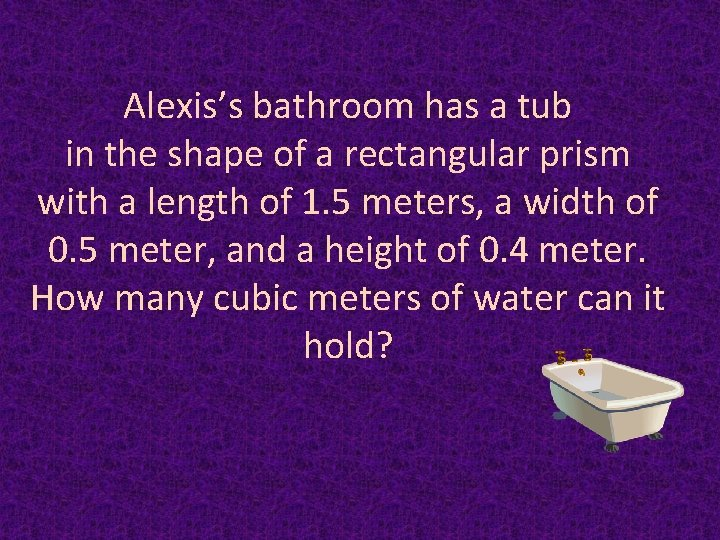Alexis’s bathroom has a tub in the shape of a rectangular prism with a length of 1. 5 meters, a width of 0. 5 meter, and a height of 0. 4 meter. How many cubic meters of water can it hold?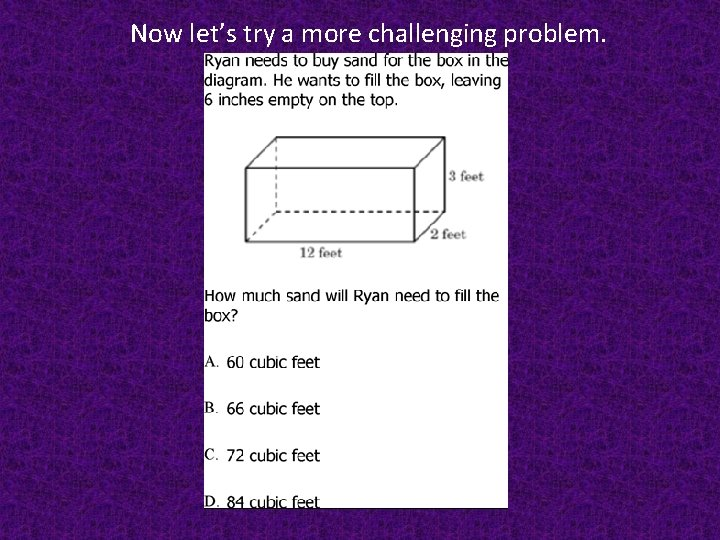Now let’s try a more challenging problem.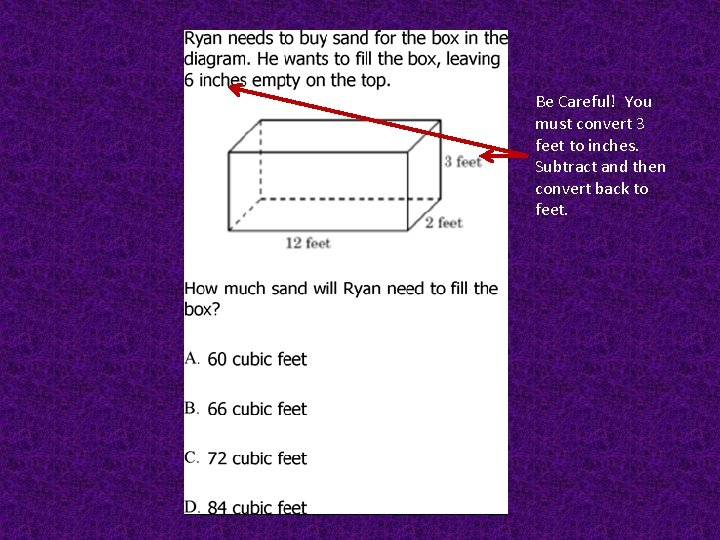Be Careful! You must convert 3 feet to inches. Subtract and then convert back to feet.Length x Width x Height Length = 12 feet Width = 2 feet Height = 30 inches or 2 ½ feet A Length x Width x Height 12 x 2 ½ 24 x 2 ½ 60BINGO Let’s Practice finding Volume